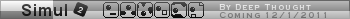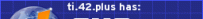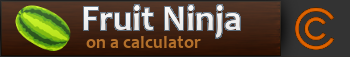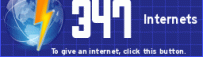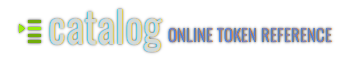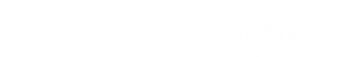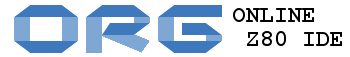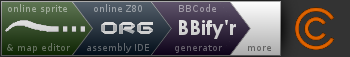###Author Topic: Point-line based collision detection/reactions [Explanation]  (Read 4445 times)

0 Members and 1 Guest are viewing this topic.

####ZippyDee

••• Posts: 729
• Rating: +83/-8
• Why not zoidberg?##### Point-line based collision detection/reactions [Explanation]
« on: March 31, 2011, 06:37:46 pm »
Howdy-doody-doodle-doo?
Collision detection and collision reactions are something game developers are constantly having to find ways to do. I'm going to explain the basic calculations needed to write efficient point-to-line collision reactions for all y'all who either don't know that much about physics, just want to brush up on some kinetics, or are just too lazy to do the calculations yourself.

THE BASICS OF COLLISIONS

Point-to-line? What's that mean?
When I say "point-to-line" (or "point-line") I am referring to a collision in which a specified point on an object collides with a solid line.

The point:
When I say "a specified point on an object" I am referring to ANY point (P) whose coordinates lie within the boundaries of your object. Obviously calculating collision with every single point in your object would be completely absurd, so collision objects are usually made up of a few keypoints to which the collision detection is applied. Those points are usually vertices and a few points that lie within longer lines. The calculations that I talk about in this explanation would be applied to these keypoints.

When a rotational force is applied to an object, the object will want to rotate around its center of mass. Every object has a center of mass. The simplest calculation for the center of mass is to assume the mass of every point in your object is the same, then you just take the average of the x-coordinates of all the points, and the average of the y-coordinates of all the points. That gives you your center of mass (xc, yc).

The line:
The line has a much shorter explanation: It's a line segment. It has endpoints, a slope, a y intercept, and angle, etc. All you really need is the endpoints and you can find the rest.

Detecting a collision
This is just a quick and dirty explanation of how you would go about determining if there was a collision between a line and a point on an object. Sorry for the lack of actual calculations. This is more about theory.In order for a collision to take place at all, the point must be moving in some way. In order for it to move, it needs to have some sort of a vector representing velocity. Because your points are rigidly attached to this object's center of mass, the velocity of the object's center of mass can be used to represent the velocity of the point. (This doesn't take into account any velocity created by the object's rotation, but that calculation is a bit much for a lot of what we're doing, so we we'll just ignore that for now. Unless you're looking for a really, really accurate physics engine, this shouldn't have a tremendous effect on your end result.)

Using this velocity, we can calculate the trajectory (again ignoring rotation around the center of mass) of the point (P) between this frame and the next frame. The trajectory will just be a line between the current location of the point (x1, y1) and the location of the point (x2, y2). From there we can calculate the equation of the line segment between those two points and check if that line segment intersects with the line segment we're testing for collision.

Line to line intersection is fairly simple:
y=m1x+b1
y=m2x+b2
Then you solve the parametric equation for x and y to find the collision.

You can also find the intersection point of two lines (not line segments) by finding the determinants of a series of matrices (which I won't really explain the specifics of at this time) and you can simplify it to a single equation as shown in pseudocode here:
Code: [Select]
function intersect(x1,y1,x2,y2,x3,y3,x4,y4){ x = ((x1*y2-y1*x2)*(x3-x4)-(x1-x2)*(x3*y4-y3*x4))/((x1-x2)*(y3-y4)-(y1-y2)*(x3-x4)) y = ((x1*y2-y1*x2)*(y3-y4)-(y1-y2)*(x3*y4-y3*x4))/((x1-x2)*(y3-y4)-(y1-y2)*(x3-x4)) return Point(x,y)}
If one of the lines is vertical, then you can simply find the x of that line and solve the equation of the other line for y at that x coordinate.

Note that those will find the intersection point of two LINES, not line-SEGMENTS. In order to correct this, you just need to check whether the point of intersection is between the start and end points of both line segments. If so, a collision occurred between the current frame and the next frame.

NOTE: When doing the collision detection calculations, it is best to calculate the collisions of all keypoints on your object, and figure out which collision happened first. Then calculate the reaction based on that collision. Otherwise it can get messy if you object is moving at a high speed and multiple reactions are being calculated at once, when they really wouldn't have happened if you had calculated in order of time. Calculating the time of the collision is simple enough:
Code: [Select]
//where (x1,y1) and (x2,y2) are the start and end points (respectively) of the point's trajectory//and (x,y) is the point of collisiontime = sqrt((x-x1)^2-(y-y1)^2)/sqrt((x2-x1)^2-(y2-y1)^2)Assuming we already know that (x,y) lies on the line defined by (x1,y1)(x2,y2), this equation will return a value between 0 and 1. 0 means the objects are colliding on the current frame (which should have been handled during the last calculation) and 1 means they will collide exactly on the next frame. Anything in between means the collision occurred somewhere between the two frames.

For anyone programming in a language such as Axe or ASM that doesn't directly use decimals, think of the time value as a percent rather than 0 to 1. So instead of 1 you use a large integer value for 100%, and 0 for 0%.

COLLISION REACTIONS

Finally the good stuff. And by good, I mean there's a lot to cover.

A basic explanation of vector dynamics for collision objects:
"Vector dynamics" is pretty much a fancy way of saying "how objects move." A vector, for those who don't know, has two properties: a magnitude, and a direction. For example, velocity is a vector: its magnitude is the speed the object is traveling at, and its direction is...well, the direction the object is traveling.

Let's talk a bit about the properties of a moving object. There are many properties that we could talk about, but there are a few that we actually care about for these purposes:
• Mass (we'll use 1 for almost all calculations just for the sake of simplicity.)
• Position (relative to the center of mass)
• Linear velocity
• Linear acceleration
• Angular velocity
• Angular acceleration
• Moment of inertia (sort of...)
Hopefully you already know what most of those are, but I'll just briefly go over them all, just in case.
-Mass is mass. It's the mass of the object, and it is represented by the mass. All clear? Good! Moving on...
-Position is simply the x and y of the center of mass of the object. As I said earlier, the center of mass can be found by averaging all the x/y points within the bounds of the object....or just average the x and y of your extremes, because we're using a constant particle mass.
-Linear velocity, or just 'velocity', is simply the speed and direction in which the object is currently moving. If it's not moving, it has no velocity. The easiest way to express velocity in programming is by using individual xVel and yVel values.
-Linear acceleration, or just 'acceleration', is the rate at which the linear velocity changes from one frame to another. We'll also express this using individual xAccel and yAccel values.
-Angular velocity, also known as 'rotational velocity', is the speed at which the object is rotating. Like linear velocity, angular velocity is also a vector, it's what's called a "rotary vector." The magnitude is the speed, and the direction is the axis it rotates about. But we'll be using the center of mass as a rotational axis, so we only need to worry about the speed, which we'll call rotVel (for 'rotational velocity').
-Angular acceleration, like regular acceleration, is the rate at which the angular velocity changes from one frame to the next. We'll express this as rotAccel.
-Moment of inertia is probably a new term for you if you haven't taken a physics class. Put simply, Inertia is an object's resistance to a change in motion (Newton's First Law: Every body remains in a state of constant velocity unless acted upon by an external unbalanced force). The Moment if Inertia is its resistance to a change in rotational motion.

When I say "Moment of inertia (sort of...)" I mean that using the exact moment of inertia is not always possible in all situations. The calculation for moment of inertia requires that you integrate over the entire surface of the object the distance to the axis of rotation squared times the mass for each particle. This is not always a simple calculation if you're using irregular shapes. In that case we can cheat and use a more regular shape that is similar. Here's a list of the moments of inertia for many regular shapes: http://en.wikipedia.org/wiki/List_of_moments_of_inertia

For this example, I'll be using a "Thin rectangular plate of height h and of width w and mass m" (as defined in that wikipedia list), so our moment of inertia (usually represented as I) calculation will be (mass*(h*h+w*w))/12. For most calculations we'll use a mass of 1, because that makes things easy, but this answer will almost definitely have to be scaled down quite a bit in order for it to look good in our pseudo-physics environment.

Bodies colliding:
Okay, so now we finally have collision, let's take a look at what's going on.

As we know, change occurs when a force is applied to an object. Physics states that Force=mass*acceleration, but because we're using a mass of 1, we can say that Force=acceleration. Remember that acceleration is also a vector, so it must have magnitude and direction.

An object has a net force Fnet acting upon it at any given time. "Net force" is as simple as the sum of all individual forces acting upon the object. From this point onward, please just understand that due to our mass being 1, any time we talk about a "force" being applied, it means an "acceleration" of equal magnitude is applied.

Gravity is a force that is acting on anything with mass at all times. So you can always assume your object has the force of gravity being applied straight down. Also, we know that "for every action there is an equal and opposite reaction." So, if an object is sitting on flat ground, the object has the force of gravity pulling it down against the ground, and the ground pushes back with an equal force in the opposite direction. For any surface of any angle, this force will be applied in a direction perpendicular to the angle of the ground. This perpendicular force is called the Normal Force (represented by FNorm).

Let's look at some simple physics: a box is placed on an inclined plane at angle θ.Gravity is pulling downward (as usual) with a force of Fg. The magnitude of the normal force can be calculated by FNorm=mass*Fg*cos(θ). But that only accounts for cos(θ) of the force of gravity, so the object would still be accelerating straight down by Fgsin(θ), meaning it would fall through the surface of the plane! That's because when FNorm is applied, the rest of the force of gravity is diverted to go PARALLEL to the surface of the plane. The magnitude of that parallel force can be calculated by F||=Fg*sin(θ).

Okay, so now the box is sliding down the inclined plane at a velocity increasing by F|| every frame. At least, it WOULD if the plane was made out of some sort of friction-less material. But since lack of friction can be assumed physically impossible, let's make this more realistic and add some in. The force of friction (Ffric) is applied in the OPPOSITE direction of F||, with a magnitude found by Ffric=FNorm. Piece of cake, right? But wait...what's that "µ" symbol doing there? The Greek letter µ ("mu", pronounced "mew") represents the coefficient of friction. Oh, dear! What does THAT mean? µ is the ratio of the force of friction between two bodies, and the force pushing the two bodies together. µ changes based on what materials the two bodies are made of. In this case, FNorm is the force pushing the two bodies together.

Wow, all this stuff with µ is getting really complex. Isn't there an easier way? Yep! Let's forget all about that µ stuff and assume that our materials will never change, so we can just make up a value to represent our µ. Just for the sake of examples, let's say our µ is 0.15. Based on that, we can say that Ffric=FNorm*0.15 and it is applied in the opposite direction as F||. If it's the exact opposite direction, why not just subtract Ffric from F||? Our new equation for the parallel force with friction applied will be F||=Fg*sin(θ)-FNorm*-0.15.

Just to make sure we're on the same page now...
FNorm=Fg*cos(θ)[
F||=Fg*sin(θ)-FNorm*0.15[/sub]
(remember that 0.15 is just the example value for µ. Play around with it until you find something you like.)

Okay, so now we know the normal force and the parallel force applied to an object on a plane that at the angle θp. Let's look at what those forces mean for us.

Let's say a box is now dropped at an angle of 0 degrees from some height onto an inclined plane at angle θp. The corner of the box will hit the plane first, so that's where the collision is processed. FNorm is now applied to the box at the point of collision Pc. This will cause the box to rotate, but we need to know how much it will rotate. Let's take a look:

First we look at the angle θa between Pc and the box's center of mass Pm. Now we look at FNorm and find the angle that it is applied at (θN). The difference between these two angles (θc) is what we'll be looking at. Using this angle we can calculate both the linear and angular acceleration on the object.
accel=FNorm*cos(θc)
But remember we're representing acceleration with xAccel and yAccel, so we have to use convert it to x and y.
xAccel=xAccel+accel*cos(θa)
yAccel=yAccel+accel*sin(θa)

The reason we use θa is that the force is applied to the center of mass, not just this specific point.
But now that only accounts for cos(θc of the force. The other sin(θc) turns into angular acceleration.
rotAccel=rotAccel+FNorm*sin(θc)

Now we've dealt with what happens with FNorm, but what about F||? That force is still applied, but now we have to do the same calculations to it as we did to the normal.
First we take the difference between θa and θ|| (we'll call it θd). Now we apply F|| to the linear and angular accelerations the same way.
accel||=F||*cos(θd)
xAccel=xAccel+acceld*cos(θa)
yAccel=yAccel+acceld*sin(θa)
rotAccel=rotAccel+F||*sin(θd)

So let's look at what we have now:
accel=FNorm*sin(θc)
xAccel=xAccel+accel*cos(θa)+acceld*cos(θa)
yAccel=yAccel+accel*sin(θa)+acceld*sin(θa)
rotAccel=rotAccel+FNorm*sin(θc)+F||*sin(θd)

I know this is still incomplete, but I thought I'd update it to at least this much and I'll update again once the full explanation is done. After that I will add graphics for explanations (which will help a lot, I'm sure) and then I'll put together an example of implementation of all of this for the final version. Thanks for your patience!
« Last Edit: April 02, 2011, 03:16:04 pm by ZippyDee »

Pushpins 'n' stuff...####DJ Omnimaga##### Re: Point-line based collision detection/reactions [Explanation]
« Reply #1 on: March 31, 2011, 06:42:58 pm »
Oh nice, another collision detection tutorial. Unfortunately Id o not have time to read it yet, but one suggestion I would like to make so far is to add pseudo-code examples, like Ashbad mentionned on IRC, and most importantly, visual examples, since some people understand better with images than just text.

####ZippyDee

••• Posts: 729
• Rating: +83/-8
• Why not zoidberg?##### Re: Point-line based collision detection/reactions [Explanation]
« Reply #2 on: March 31, 2011, 06:44:44 pm »
Yeah, I'll go back and add some to the collision detection. But I'll have multiple visual and pseudo-code examples in the collision reactions explanations.Pushpins 'n' stuff...####ZippyDee

••• Posts: 729
• Rating: +83/-8
• Why not zoidberg?##### Re: Point-line based collision detection/reactions [Explanation]
« Reply #3 on: April 01, 2011, 09:43:46 pm »
Damn, this is ending up being really long, and it'll be even longer once I add visuals and implementations. Anyway, I'll bump what I have for now.

Pushpins 'n' stuff...####Deep Toaster

• So much to do, so much time, so little motivation
• LV13 Extreme Addict (Next: 9001)
••• Posts: 8217
• Rating: +758/-15##### Re: Point-line based collision detection/reactions [Explanation]
« Reply #4 on: April 01, 2011, 11:10:32 pm »
Wow, this is advanced stuffAnd useful too. I think I really will be using some of it. Thanks ZippyDee!

Damn, this is ending up being really long, and it'll be even longer once I add visuals and implementations. Anyway, I'll bump what I have for now.

Nah, I've seen (and posted) longerYou have a lot of detailed explanations though. And as a suggestion, some graphics would make it easier to understand